# Diagramming Atoms Labeling Electrons Part 1 Supplement to

• Slides: 14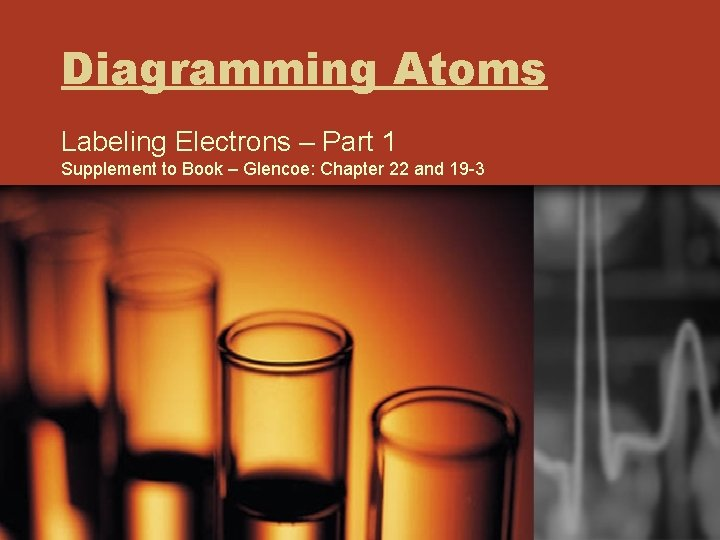Diagramming Atoms Labeling Electrons – Part 1 Supplement to Book – Glencoe: Chapter 22 and 19 -3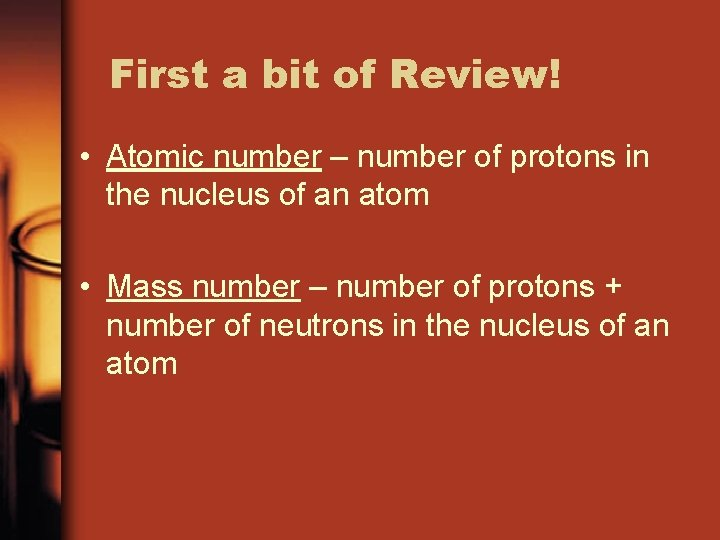First a bit of Review! • Atomic number – number of protons in the nucleus of an atom • Mass number – number of protons + number of neutrons in the nucleus of an atom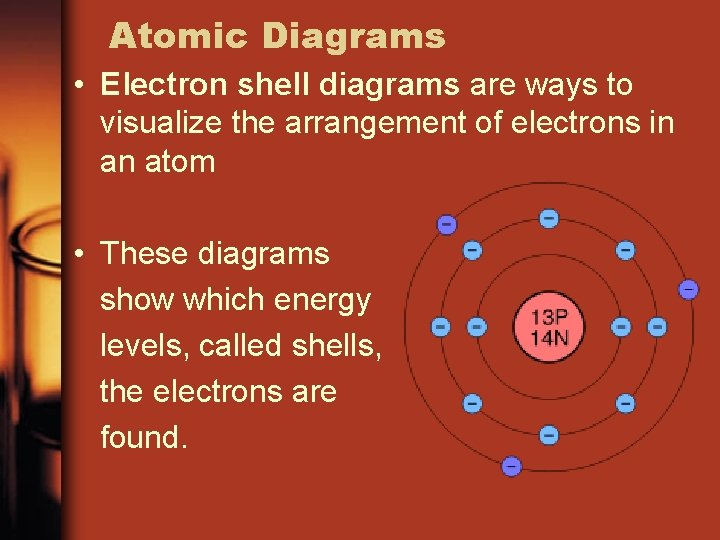Atomic Diagrams • Electron shell diagrams are ways to visualize the arrangement of electrons in an atom • These diagrams show which energy levels, called shells, the electrons are found.Electron shell diagrams Particle locations 1. Nucleus - All protons (p+) are found in the nucleus - All neutrons (N) are found in the nucleus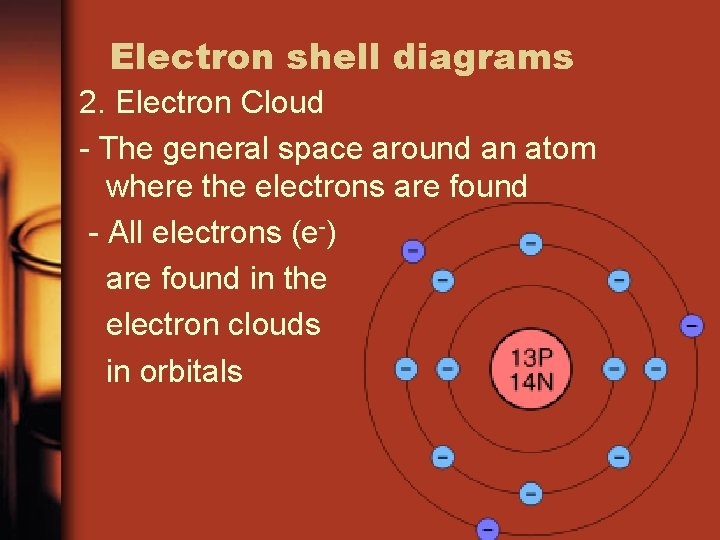Electron shell diagrams 2. Electron Cloud - The general space around an atom where the electrons are found - All electrons (e-) are found in the electron clouds in orbitals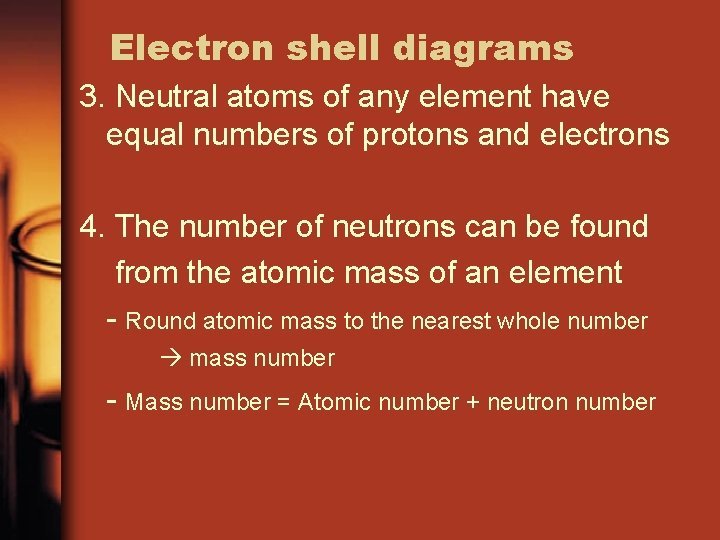Electron shell diagrams 3. Neutral atoms of any element have equal numbers of protons and electrons 4. The number of neutrons can be found from the atomic mass of an element - Round atomic mass to the nearest whole number mass number - Mass number = Atomic number + neutron numberEnergy levels in atoms Quantum Numbers – values which represent theoretical “address” of an electron in an atom 1. Electrons are modeled in atoms combining two atomic models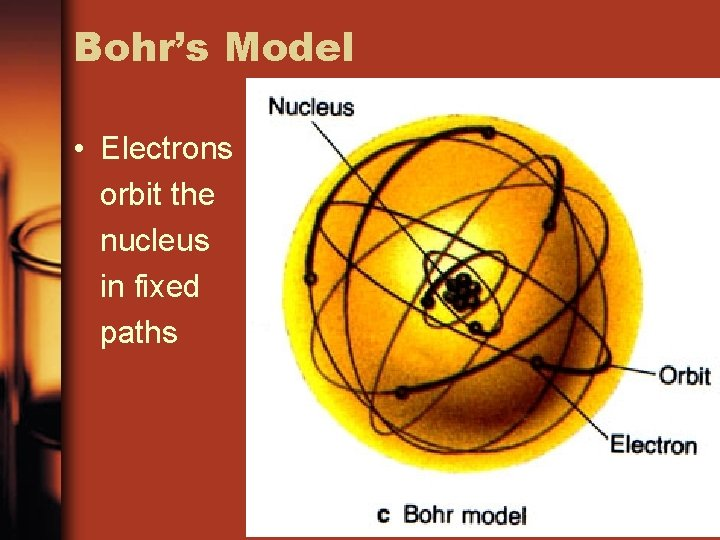Bohr’s Model • Electrons orbit the nucleus in fixed paths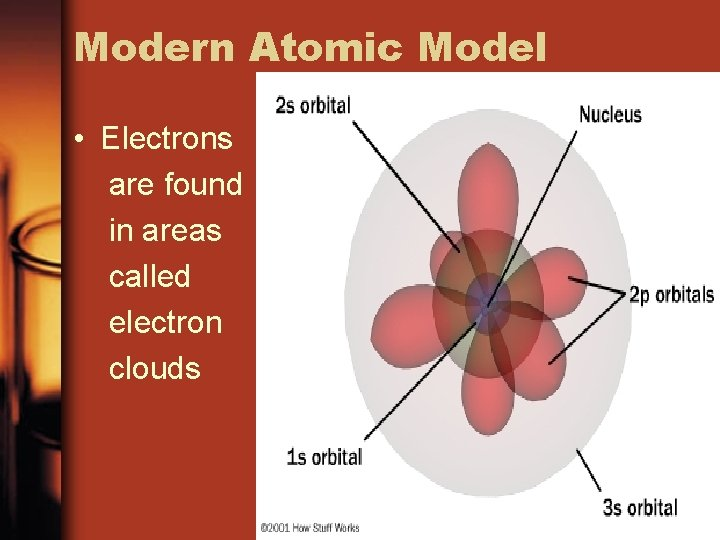Modern Atomic Model • Electrons are found in areas called electron clouds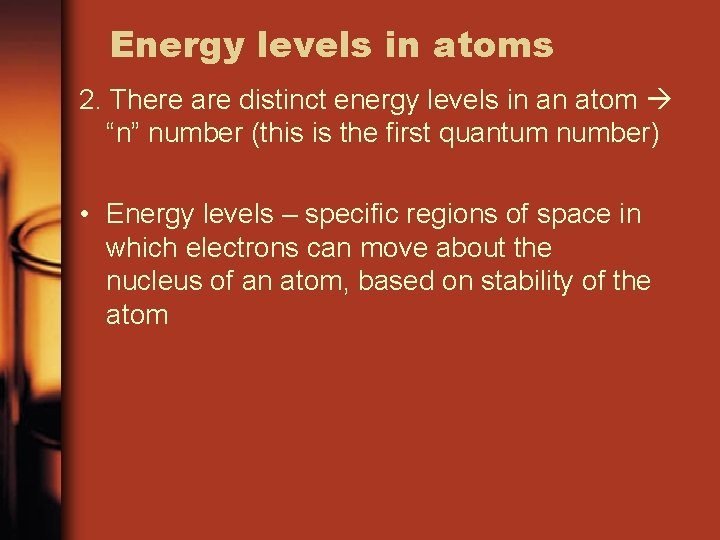Energy levels in atoms 2. There are distinct energy levels in an atom “n” number (this is the first quantum number) • Energy levels – specific regions of space in which electrons can move about the nucleus of an atom, based on stability of the atom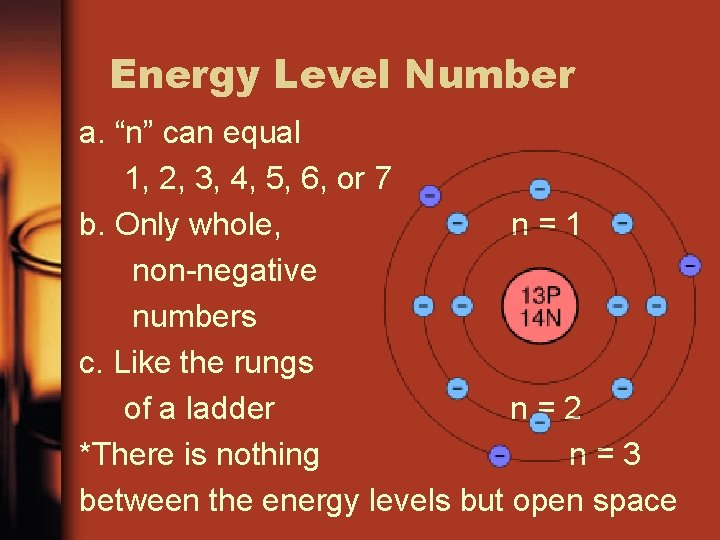Energy Level Number a. “n” can equal 1, 2, 3, 4, 5, 6, or 7 b. Only whole, n=1 non-negative numbers c. Like the rungs of a ladder n=2 *There is nothing n=3 between the energy levels but open space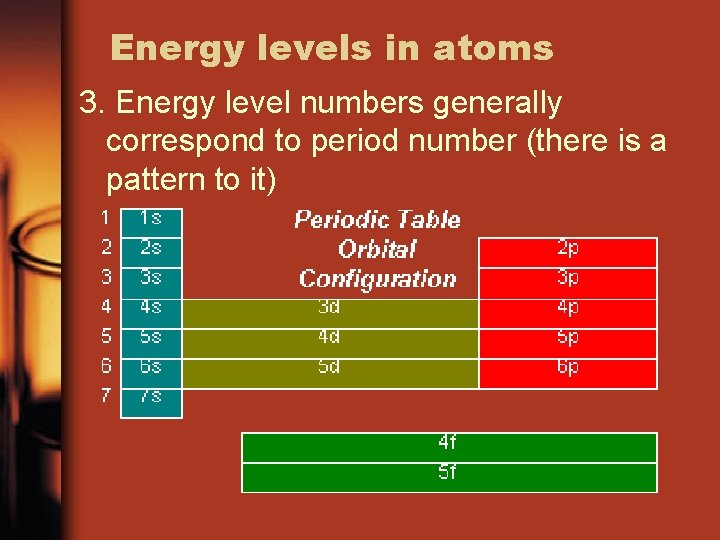Energy levels in atoms 3. Energy level numbers generally correspond to period number (there is a pattern to it)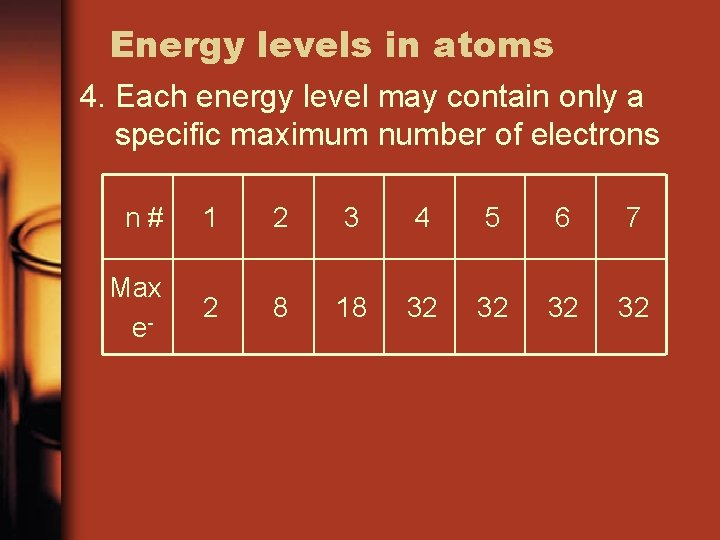Energy levels in atoms 4. Each energy level may contain only a specific maximum number of electrons n# 1 2 3 4 5 6 7 Max e- 2 8 18 32 32Energy levels in atoms 5. Energy levels fill up in a specific order lowest energy (n = 1) (most stable) to highest energy (n = 7) (least stable)